# Measuring lengths with different units

##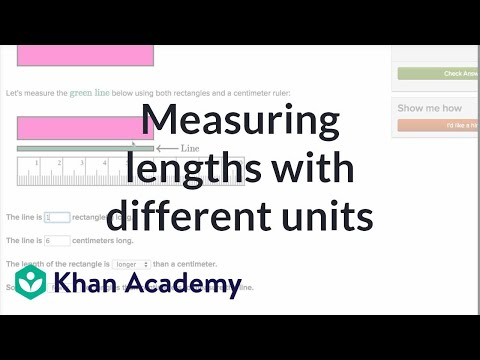By Khan Academy

Measuring lengths with different units# Measuring lengths 2

##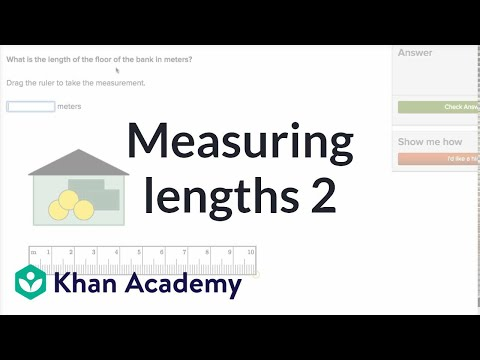By Khan Academy

Measuring lengths 2# Measuring lengths 1

##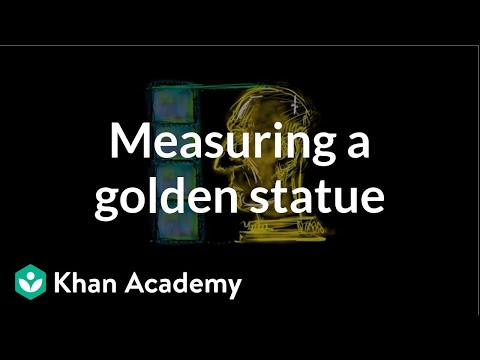By Khan Academy

Measure an object with same-size length units that span it with no gaps or overlaps.# Measure line segments

##By MathPlanetVideos

Measure the length of the line segment.# Units of Measure | Good To Know | WSKG

##By WSKG Public Media

metric system to measure length, mass, and capacity.# Law of cosines

##By Khan Academy

Sal is given a triangle with all side lengths but no angle measure, and he finds one of the angle measures using the law of cosines.# [3.MD.4-1.0] Visual Representations of Data - Common Core Standard

##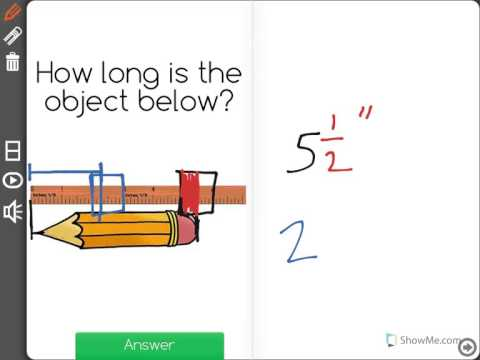By Freckle education

Generate measurement data by measuring lengths using rulers marked with halves and fourths of an inch.# Law of cosines

##By Khan Academy

Sal is given a triangle with all side lengths but no angle measure, and he finds one of the angle measures using the law of cosines.# 15.2 Inequalities in a Triangle (Lesson)

##By AutenMath

A lesson on the inequality theorems and relationships of side length and angle measures in a triangle# Solving similar triangles 1

##By Khan Academy

Sal solves two problems where a missing side length is found by proving that triangles are similar and using this to find the measure.# Solving similar triangles 2

##By Khan Academy

Sal solves two problems where a missing side length is found by proving that triangles are similar and using this to find the measure.# Radian and degree | Unit circle definition of trig functions | Trigonometry | Khan Academy

##By Khan Academy

This video explains what a radian is and how we determine the radian measure of an angle. The key point here is that the radian measure of an angle is the length of the arc (in radius lengths) cut off by that angle (if the angle's vertex is on the center of the circle). You may ask: Won't the size of the circle affect the radian measure of an angle? A key feature of circles is that they are all similar (and thus, proportional). When we account for their larger size, we take into account the larger radius. As a result, the radian measure is the same for all circles (since the radian measure is how many radius lengths long the arc is).# Measuring to the Nearest Quarter inch

##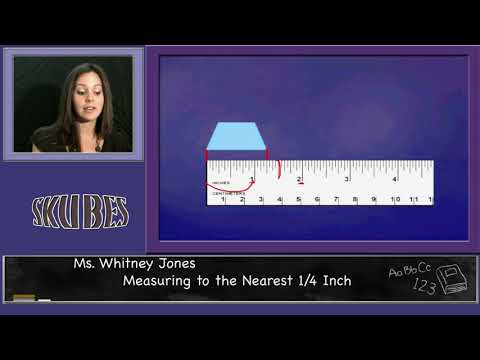By Skubes ed

Generate measurement data by measuring lengths using rulers marked with halves and fourths of an inch. Show the data by making a line plot, where the horizontal scale is marked off in appropriate units— whole numbers, halves, or quarters.# Line Plot Showing Measurement Data

##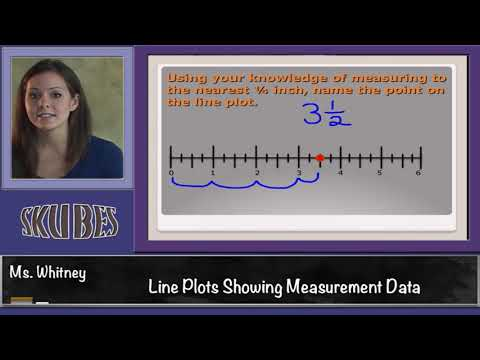By Skubes ed

Generate measurement data by measuring lengths using rulers marked with halves and fourths of an inch. Show the data by making a line plot, where the horizontal scale is marked off in appropriate units— whole numbers, halves, or quarters.# Measuring Area in Square Units

##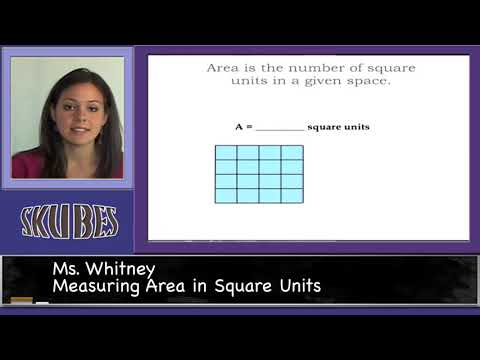By Skubes ed

. A square with side length 1 unit, called “a unit square,” is said to have “one square unit” of area, and can be used to measure area.# Law of sines

##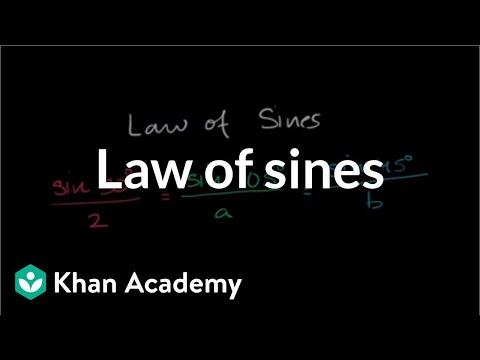By Khan Academy

Sal is given a triangle with two angle measures and one side length, and he finds all the missing side lengths and angle measuresï¿½ï¿½ï¿½ï¿½ï¿½ï¿½ï¿½ï¿½ï¿½ï¿½ï¿½ï¿½using the law of sines.# Law of sines

##By Khan Academy

Sal is given a triangle with two angle measures and one side length, and he finds all the missing side lengths and angle measuresï¿½ï¿½ï¿½ï¿½ï¿½ï¿½ï¿½ï¿½ï¿½ï¿½ï¿½ï¿½using the law of sines.# Law of cosines

##By Khan Academy

Sal is given a triangle with two side lengths and the contained angle measure, and he finds the missing side lengthï¿½ï¿½ï¿½ï¿½ï¿½ï¿½ï¿½ï¿½ï¿½ï¿½ï¿½ï¿½using the law of cosines.# Law of cosines

##By Khan Academy

Sal is given a triangle with two side lengths and the contained angle measure, and he finds the missing side lengthï¿½ï¿½ï¿½ï¿½ï¿½ï¿½ï¿½ï¿½ï¿½ï¿½ï¿½ï¿½using the law of cosines.# Metric Unit Conversions - YourTeacher.com - Pre Algebra Help

##By yourteachermathhelp

Students learn that in the metric system all measures of length are based on meters. For example a centimeter is 1/100 of a meter. And all measures of weight are based on grams. For example a kilogram is 1000 grams. And all measures of volume are based on liters. For example a milliliter is 1/1000 of a liter. Students are then asked to solve problems using conversion factors such as 4 500 milligrams = ____ grams.